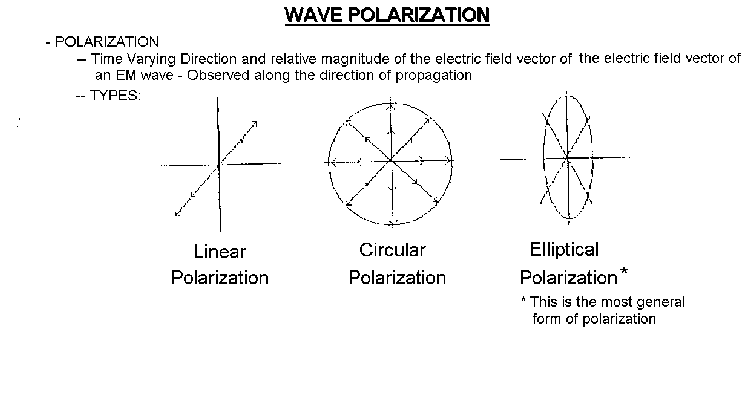## B. The Purpose of each polarization

Having discussed the criteria used to select the frquencies of the SSMI, the discussion will now turn to the utility of the cross polarization channels at 19, 37, and 85 GHz.

The manner in which we measure radiation might depend not only on the phenomena which emits it, but also on the spatial orientation in which it is emitted. Basically, the emissivity of an object or surface may be a function of polarization of the radiation. As stated in Grant ,

"Measurement of the amount of polarization of the emitted can be performed by using
two channels of the same frequency, one of which detects vertically polarized energy
while the other detects horizontally polarized energy. Usually an EM [electromagnetic]
wave is not polarized purely in the vertical or horizontal plane. In fact, it may not be
polarized at all. In this case, both the vertical channel and the horizontal channel will
each detect part of the energy from the wave. If the wave is closer to being vertically
polarized than horizontally polarized, then the vertical channel will detect the most
energy. The difference between the brightness of an object on the vertical channel and in
he horizontal channel provides useful infor- -mation about it."

There are four types of electromagnetic [EM] polarization that we are concerned with: Random Polarization, Linear Polarization, Circular Polarization, and Elliptical Polarization (see examples in figure 9). In random polarization, there is no preferred sense of polarization. In linear polarization, the E vector remains in a single plane. Two special cases of linear polarization are the horizontal and vertical polarization. "Horizontal polarization is .. defined as the state where the electric vector is perpendicular to the plane of incidence. The vertical polarization corresponds to the case where the electric vector is in the plane of incidence. " [Elachi, 1987]. Elliptical polarization is a case where two waves of the same frequency , different phase, different amplitude, and different polarization combine into a single wave. The result is the vector addition of the two E vectors. The combined E vector will seem to rotate in the pattern of an ellipse about the vector forming the direction of motion. Circular polarization is just a special case of elliptical polarization, where all the above holds true, except that the amplitudes are now both the same. The final electric field vector [E] precesses around the axis of motion in a circle.

##### -### Figure 9

##### WAVE POLARIZATION

The emissions at 22 GHz come from atmospheric water vapor. Such emissions are usually randomly polarized, so it is not necesary to measure the horizontal polarization. This is the reason for only one vertical channel at 22 GHz on the SSMI.

The other channels will experience different phenomenon that make polarimetry important. For example, Sea ice exhibits some distinctive differences in polarization at 85 GHz. Desert regions also display polarization differences at many frequencies. The work of Barrett  observed:

"Polarization of reflected, transmitted and emitted PM [passive microwave] radiation
depends on molecular or cystalline properties and the surface roughness of a medium.
Like emissivity, it is a function of angle of view. Water, having a high dielectric constant
produces highly polarized radiation at oblique viewing angles : for example, at 37 GHz ,
calculated brightness temperature differences between horizontally and vertically